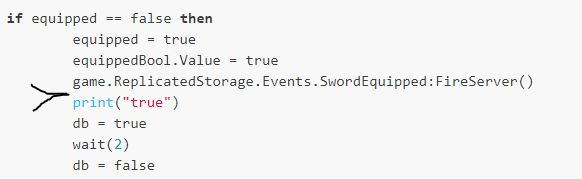# Why i can not make my animation run

Hello, i have a problem in my script. The problem is I want add play animation command to my script. I used The Dev King’s tutorial but it did not work.

Here is code:

``````local UIS = game:GetService("UserInputService")
local db = false
local equipped = false
local equippedBool = game.ReplicatedStorage.Events.Equipped

UIS.InputBegan:Connect(function(input)

if input.KeyCode == Enum.KeyCode.E then
if db == false then

if equipped == false then
equipped = true
equippedBool.Value = true
game.ReplicatedStorage.Events.SwordEquipped:FireServer()
print("true")
db = true
wait(2)
db = false
else
equipped = false
equippedBool.Value = false
game.ReplicatedStorage.Events.SwordUnEquipped:FireServer()
print("false")
db = true
wait(2)
db = false
end
end
end
end)
``````

I would be happy if you tell me how can i make the animation play here(marked place):``````Local track = character.Humanoid:LoadAnimation(path to animation)
Track:Play()``````

Put an “Animation” as a direct child of the script. Insert the animation Id into the animation.

``````local player = game.Players.LocalPlayer --top line of script
local animation = script.Animation

--later in code